xGear trainEncyclopedia
A gear train is formed by mounting gear
Gear
A gear is a rotating machine part having cut teeth, or cogs, which mesh with another toothed part in order to transmit torque. Two or more gears working in tandem are called a transmission and can produce a mechanical advantage through a gear ratio and thus may be considered a simple machine....

s on a frame so that the teeth of the gears engage. Gear teeth are designed to ensure the pitch circles of engaging gears roll on each other without slipping, this provides a smooth transmission of rotation from one gear to the next.

The transmission of rotation between contacting toothed wheels can be traced back to the Antikythera mechanism
Antikythera mechanism
The Antikythera mechanism is an ancient mechanical computer designed to calculate astronomical positions. It was recovered in 1900–1901 from the Antikythera wreck. Its significance and complexity were not understood until decades later. Its time of construction is now estimated between 150 and 100...

of Greece and the South Pointing Chariot
South Pointing Chariot
The south-pointing chariot was an ancient Chinese two-wheeled vehicle that carried a movable pointer to indicate the south, no matter how the chariot turned. Usually, the pointer took the form of a doll or figure with an outstretched arm...

of China. Illustrations by the renaissance scientist Georgius Agricola show gear trains with cylindrical teeth. The implementation of the involute tooth
Involute gear
The involute gear profile is the most commonly used system for gearing today. In an involute gear, the profiles of the teeth are involutes of a circle. In involute gear design contact between a pair of gear teeth occurs...

yielded a standard gear design that provides a constant speed ratio.

Some important features of gears and gear trains are:
• The ratio of the pitch circles of mating gears defines the speed ratio
Gear ratio
The gear ratio of a gear train is the ratio of the angular velocity of the input gear to the angular velocity of the output gear, also known as the speed ratio of the gear train. The gear ratio can be computed directly from the numbers of teeth of the various gears that engage to form the gear...

Mechanical advantage is a measure of the force amplification achieved by using a tool, mechanical device or machine system. Ideally, the device preserves the input power and simply trades off forces against movement to obtain a desired amplification in the output force...

of the gear set.
• A planetary gear train
Epicyclic gearing
Epicyclic gearing or planetary gearing is a gear system consisting of one or more outer gears, or planet gears, revolving about a central, or sun gear. Typically, the planet gears are mounted on a movable arm or carrier which itself may rotate relative to the sun gear...

provides high gear reduction in a compact package.
• It is possible to design gear teeth for gears that are non-circular
Non-circular gear
A non-circular gear is a special gear design with special characteristics and purpose. While a regular gear is optimized to transmit torque to another engaged member with minimum noise and wear and with maximum efficiency, a non-circular gear's main objective might be ratio variations, axle...

, yet still transmit torque smoothly.
• The speed ratios of chain
Chain drive
Chain drive is a way of transmitting mechanical power from one place to another. It is often used to convey power to the wheels of a vehicle, particularly bicycles and motorcycles...

and belt drives
Belt (mechanical)
A belt is a loop of flexible material used to link two or more rotating shafts mechanically. Belts may be used as a source of motion, to transmit power efficiently, or to track relative movement. Belts are looped over pulleys. In a two pulley system, the belt can either drive the pulleys in the...

are computed in the same way as gear ratios. See bicycle gearing
Bicycle gearing
A bicycle gear or gear ratio refers to the rate at which the rider's legs turn compared to the rate at which the wheels turn. Bicycle gearing refers to how the gear ratio is set or changed. On some bicycles, there is only one gear so the ratio is fixed. Most modern bicycles have multiple gears,...

.

Gear teeth are designed so that the number of teeth on a gear is proportional to the radius of its pitch circle, and so that the pitch circles of meshing gears roll on each other without slipping. The speed ratio
Gear ratio
The gear ratio of a gear train is the ratio of the angular velocity of the input gear to the angular velocity of the output gear, also known as the speed ratio of the gear train. The gear ratio can be computed directly from the numbers of teeth of the various gears that engage to form the gear...

for a pair of meshing gears can be computed from ratio of the radii of the pitch circles and the ratio of the number of teeth on each gear.
The velocity v of the point of contact on the pitch circles is the same on both gears, and is given by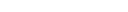where input gear A has radius rA and meshes with output gear B of radius rB,
therefore,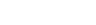where NA is the number of teeth on the input gear and NB is the number of teeth on the output gear.

Mechanical advantage is a measure of the force amplification achieved by using a tool, mechanical device or machine system. Ideally, the device preserves the input power and simply trades off forces against movement to obtain a desired amplification in the output force...

of a pair of meshing gears for which the input gear has NA teeth and the output gear has NB teeth is given by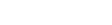This shows that if the output gear GB has more teeth than the input gear GA, then the gear train amplifies the input torque. And, if the output gear has fewer teeth than the input gear, then the gear train reduces the input torque.

If the output gear of a gear train rotates more slowly than the input gear, then the gear train is called a speed reducer. In this case, because the output gear must have more teeth than the input gear, the speed reducer will amplify the input torque.

## Analysis using virtual work

For this analysis, we consider a gear train that has one degree-of-freedom, which means the angular rotation of all the gears in the gear train are defined by the angle of the input gear.

The size of the gears and the sequence in which they engage define the ratio of the angular velocity ωA of the input gear to the angular velocity ωB of the output gear, known as the speed ratio, or gear ratio
Gear ratio
The gear ratio of a gear train is the ratio of the angular velocity of the input gear to the angular velocity of the output gear, also known as the speed ratio of the gear train. The gear ratio can be computed directly from the numbers of teeth of the various gears that engage to form the gear...

, of the gear train. Let R be the speed ratio
Gear ratio
The gear ratio of a gear train is the ratio of the angular velocity of the input gear to the angular velocity of the output gear, also known as the speed ratio of the gear train. The gear ratio can be computed directly from the numbers of teeth of the various gears that engage to form the gear...

, then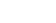The input torque TA acting on the input gear GA is transformed by the gear train into the output torque TB exerted by the output gear GB. If we assume, that the gears are rigid and that there are no losses in the engagement of the gear teeth, then the principle of virtual work can be used to analyze the static equilibrium of the gear train.

Let the angle θ of the input gear be the generalized coordinate of the gear train, then the speed ratio R of the gear train defines the angular velocity of the output gear in terms of the input gear, that is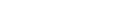The formula for the generalized force obtained from the principle of virtual work
Virtual work
Virtual work arises in the application of the principle of least action to the study of forces and movement of a mechanical system. Historically, virtual work and the associated calculus of variations were formulated to analyze systems of rigid bodies, but they have also been developed for the...

with applied torques yields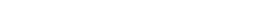Mechanical advantage is a measure of the force amplification achieved by using a tool, mechanical device or machine system. Ideally, the device preserves the input power and simply trades off forces against movement to obtain a desired amplification in the output force...

of the gear train is the ratio of the output torque TB to the input torque TA, and the above equation yields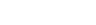Thus, the speed ratio of a gear train also defines its mechanical advantage
Mechanical advantage is a measure of the force amplification achieved by using a tool, mechanical device or machine system. Ideally, the device preserves the input power and simply trades off forces against movement to obtain a desired amplification in the output force...

. This shows that if the input gear rotates faster than the output gear, then the gear train amplifies the input torque. And, if the input gear rotates slower than the output gear, then the gear train reduces the input torque.

## Examples

Types of gear trains include:
• Simple gear train
• Compound gear train
• Epicyclic gear train
• Reverted gear train

• Wheel train (horology)
Wheel train (horology)
In horology, a wheel train is the gear train of a mechanical watch or clock. Although the term is used for other types of gear trains, the long history of mechanical timepieces has created a traditional terminology for their gear trains which is not used in other applications of gears.Watch...

• Gear
Gear
A gear is a rotating machine part having cut teeth, or cogs, which mesh with another toothed part in order to transmit torque. Two or more gears working in tandem are called a transmission and can produce a mechanical advantage through a gear ratio and thus may be considered a simple machine....

• Gear ratio
Gear ratio
The gear ratio of a gear train is the ratio of the angular velocity of the input gear to the angular velocity of the output gear, also known as the speed ratio of the gear train. The gear ratio can be computed directly from the numbers of teeth of the various gears that engage to form the gear...

• Powertrain
Powertrain
In a motor vehicle, the term powertrain or powerplant refers to the group of components that generate power and deliver it to the road surface, water, or air. This includes the engine, transmission, drive shafts, differentials, and the final drive...

Mechanical advantage is a measure of the force amplification achieved by using a tool, mechanical device or machine system. Ideally, the device preserves the input power and simply trades off forces against movement to obtain a desired amplification in the output force...

• Virtual work
Virtual work
Virtual work arises in the application of the principle of least action to the study of forces and movement of a mechanical system. Historically, virtual work and the associated calculus of variations were formulated to analyze systems of rigid bodies, but they have also been developed for the...

• Mechanism (engineering)
Mechanism (engineering)
A mechanism is a device designed to transform input forces and movement into a desired set of output forces and movement. Mechanisms generally consist of moving components such as gears and gear trains, belt and chain drives, cam and follower mechanisms, and linkages as well as friction devices...

• Machine (mechanical)
Machine (mechanical)
The mechanical properties of a machine manage power to achieve desired forces and movement. Modern machines often include computers and sensors that monitor performance and plan movement, and are called mechanical systems....

• Mechanical system
Mechanical system
A mechanical system manages power to accomplish a task that involves forces and movement. Mechanical is derived from the Latin word machina, which in turn derives from the Doric Greek μαχανά , Ionic Greek μηχανή "contrivance, machine, engine" and that from μῆχος , "means, expedient, remedy".The...